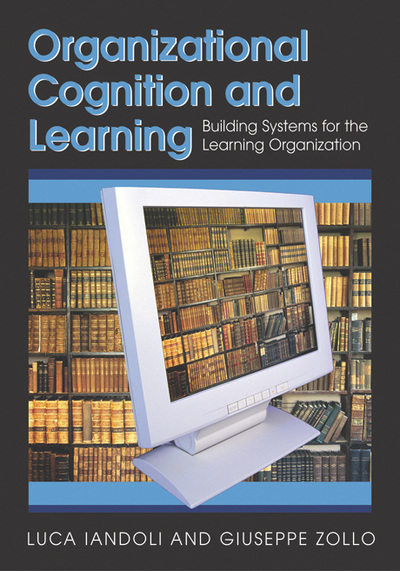# Modeling Discourses

Luca Iandoli (University of Napoli Federico II, Italy) and Giuseppe Zollo (University of Napoli Federico II, Italy)
Copyright: © 2007 |Pages: 19
DOI: 10.4018/978-1-59904-313-5.ch012
OnDemand PDF Download:
Available
\$37.50
No Current Special Offers

## Abstract

In this chapter we introduce the concept of verbal model. A verbal model is a mathematical modeling of the variables contained in a discourse and of the relationships among them. A discourse may contain linguistic variables, i.e., variables assuming linguistic values. For instance the variable “performance” can assume values such as poor, satisfying, above average, excellent, etc. Verbal models can accept linguistic inputs as well as quantitative variables and can be implemented starting from the discourse map through fuzzy logic algorithms. Such algorithms can simulate to a certain degree the theories of action contained in explanations. This chapter presents an introduction to fuzzy logic and to some possible ways of constructing verbal models, while we provide several examples in Chapters XIII and XIV.

## Complete Chapter List

Search this Book:
Reset